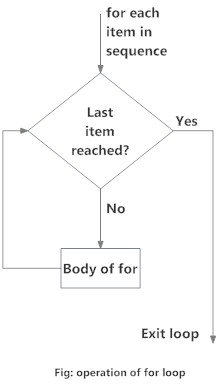# What is a for loop in Python

## What is for loop in Python?

The for loop in Python is used to iterate over a sequence (list, tuple, string) or other iterable objects. Iterating over a sequence is called traversal.

### Syntax of for Loop

```for val in sequence:
Body of for
```
Here, `val` is the variable that takes the value of the item inside the sequence on each iteration.
Loop continues until we reach the last item in the sequence. The body of for loop is separated from the rest of the code using indentation.

### Flowchart of for Loop## The range() function

We can generate a sequence of numbers using `range()` function. `range(10)` will generate numbers from 0 to 9 (10 numbers).
We can also define the start, stop and step size as `range(start,stop,step size)`. step size defaults to 1 if not provided.
This function does not store all the values in memory, it would be inefficient. So it remembers the start, stop, step size and generates the next number on the go.
To force this function to output all the items, we can use the function `list()`.
The following example will clarify this.
```# Output: range(0, 10)
print(range(10))

# Output: [0, 1, 2, 3, 4, 5, 6, 7, 8, 9]
print(list(range(10)))

# Output: [2, 3, 4, 5, 6, 7]
print(list(range(2, 8)))

# Output: [2, 5, 8, 11, 14, 17]
print(list(range(2, 20, 3))) ```
```
for loop with else

A for loop can have an optional else block as well. The `else` part is executed if the items in the sequence used in for loop exhausts.

break statement can be used to stop a for loop. In such case, the else part is ignored.```